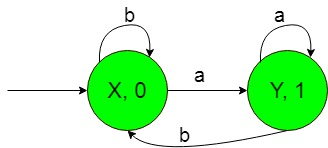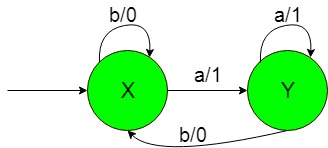Related Articles
Finite Automata with Output (Set 3)
• Last Updated : 20 Nov, 2019

Prerequisite: Mealy and Moore Machines, Difference between Mealy machine and Moore machine
In this article, we will see some designing of Finite Automata with Output i.e, Moore and Mealy machines.

Problem: Construction of the machines that take set of all string over {a, b} as input and count number of substring ‘a’.
That is here we have,
Ε = {a, b} and
Δ = {0, 1}
where Ε and Δ are the input and output alphabet respectively.
The required Moore machine is constructed below:-Explanation:
In the above diagram, the initial state ‘X’ on getting ‘b’ as the input it remains in the state of itself and print ‘0’ as the output and on getting ‘a’ as the input it transits to a state ‘Y’ and prints ‘1’ as the output. The state ‘Y’ on getting ‘a’ as the input it remains in the state of itself and prints ‘1’ as the output and on getting ‘b’ as the input it comes back to the state ‘X’ and prints ‘0’ as the output.
Thus finally above Moore machine can easily count substring ‘a’ i.e, on getting ‘a’ as the substring it gives ‘1’ as the output thus on counting number of ‘1’ we can count the number of substrings ‘a’.

The required Mealy machine is constructed below:-Explanation:
In the above diagram, the initial state ‘X’ on getting ‘b’ as the input it remains in the state of itself and print ‘0’ as the output and on getting ‘a’ as the input it transits to a state ‘Y’ and prints ‘1’ as the output. The state ‘Y’ on getting ‘a’ as the input it remains in the state of itself and prints ‘1’ as the output and on getting ‘b’ as the input it comes back to the state ‘X’ and prints ‘0’ as the output.
Thus finally above Moore machine can easily count substring ‘a’ i.e, on getting ‘a’ as the substring it gives ‘1’ as the output thus on counting number of ‘1’ we can count the number of substrings ‘a’.

Attention reader! Don’t stop learning now. Get hold of all the important CS Theory concepts for SDE interviews with the CS Theory Course at a student-friendly price and become industry ready.

My Personal Notes arrow_drop_up
Recommended Articles
Page :